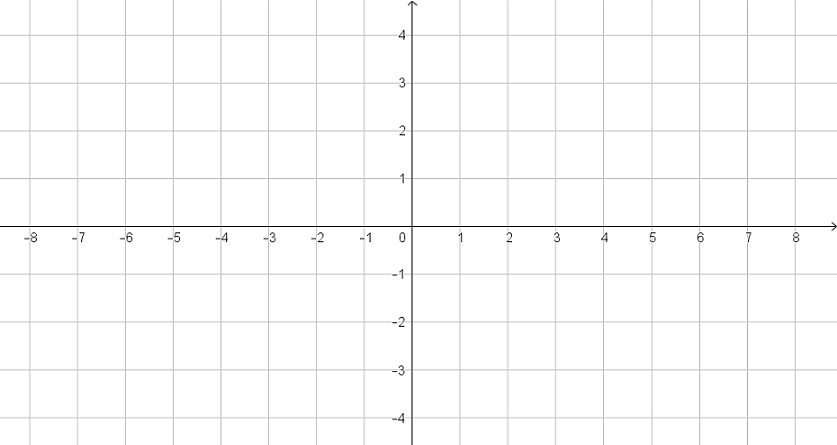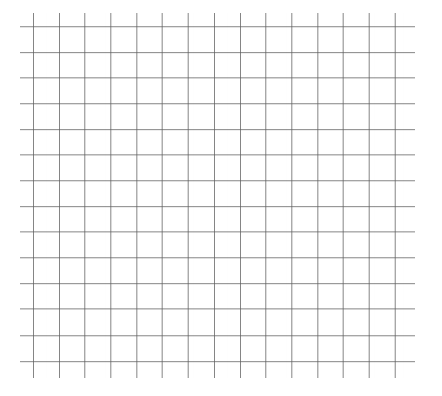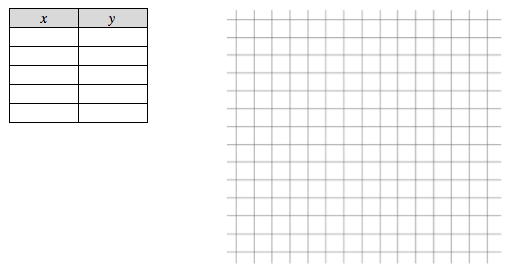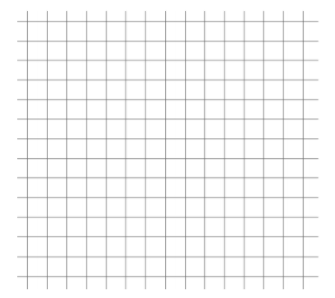# Linear Relationships

## Objective

Graph a linear equation using a table of values.

## Common Core Standards

### Core Standards

?

• 8.F.B.4 — Construct a function to model a linear relationship between two quantities. Determine the rate of change and initial value of the function from a description of a relationship or from two (x, y) values, including reading these from a table or from a graph. Interpret the rate of change and initial value of a linear function in terms of the situation it models, and in terms of its graph or a table of values.

?

• 6.EE.C.9

• 7.RP.A.2

## Criteria for Success

?

1. Create a table of values from an equation to represent solutions to the equation, using a variety of values for x including positives, negatives, and zero where applicable.
2. Use a table of values to graph a linear equation, understanding that the points connect together to form a straight line.
3. Graph linear equations in all four quadrants of the coordinate plane.
4. Understand that solutions to the equation or situation can be represented in both the table of values and on the line in the graph.

## Tips for Teachers

?

In Lesson 4, students begin to venture beyond proportional relationships and explore linear functions in all four quadrants of the coordinate plane with positive and negative slopes. They start by graphing linear equations using a table of values, a valuable skill for graphing that students had some exposure to in Unit 4 Lesson 7. In the lessons to follow, students will investigate slope and the y-intercept to find more efficient ways to graph linear equations.

#### Remote Learning Guidance

If you need to adapt or shorten this lesson for remote learning, we suggest prioritizing Anchor Problem 1 (benefits from worked example) and Anchor Problem 3 (can be done independently). Find more guidance on adapting our math curriculum for remote learning here.

#### Fishtank Plus

• Problem Set
• Student Handout Editor
• Vocabulary Package

## Anchor Problems

?

### Problem 1

After a house was built, it starts to settle into the ground. Its elevation starts at sea level, and the house sinks $\frac{1}{2}$ cm each year.

1. Write a function to represent the elevation of the house, $y$, in cm after $x$ years.
2. Create a table of values for the function with at least 5 values of $x$ and $y$.
3. Use the table of values to graph the relationship.### Problem 2

Emily tells you that she scored 18 points in a basketball game.

1. Write down all the possible ways she could have scored 18 points with only two- and three-point baskets. Use the table below to organize your work.
1. Write an equation to represent the situation, with $x$ as the number of two-point baskets and $y$ as the number of three-point baskets Emily scored. Then graph the situation.#### References

EngageNY Mathematics Grade 8 Mathematics > Module 4 > Topic B > Lesson 12Opening Exercise

Grade 8 Mathematics > Module 4 > Topic B > Lesson 12 of the New York State Common Core Mathematics Curriculum from EngageNY and Great Minds. © 2015 Great Minds. Licensed by EngageNY of the New York State Education Department under the CC BY-NC-SA 3.0 US license. Accessed Dec. 2, 2016, 5:15 p.m..

Modified by The Match Foundation, Inc.

### Problem 3

Find five solutions for the linear equation ${y=2x-10}$ to create a table of values. Use a variety of values for $x$. Plot the points and graph the situation on the coordinate plane.#### References

EngageNY Mathematics Grade 8 Mathematics > Module 4 > Topic B > Lesson 12Exploratory Challenge #2

Grade 8 Mathematics > Module 4 > Topic B > Lesson 12 of the New York State Common Core Mathematics Curriculum from EngageNY and Great Minds. © 2015 Great Minds. Licensed by EngageNY of the New York State Education Department under the CC BY-NC-SA 3.0 US license. Accessed Dec. 2, 2016, 5:15 p.m..

Modified by The Match Foundation, Inc.

## Problem Set

?The following resources include problems and activities aligned to the objective of the lesson that can be used to create your own problem set.

• Include problems where students are given a linear equation and are asked to create a table of values in order to graph the equation. Include a mix of proportional and non-proportional relationships with positive and negative slopes.

?

### Problem 1

Is the point ${(1, 3)}$ a solution to the linear equation ${5x-9y=32}$ ? Explain.

#### References

EngageNY Mathematics Grade 8 Mathematics > Module 4 > Topic B > Lesson 12Exit Ticket

Grade 8 Mathematics > Module 4 > Topic B > Lesson 12 of the New York State Common Core Mathematics Curriculum from EngageNY and Great Minds. © 2015 Great Minds. Licensed by EngageNY of the New York State Education Department under the CC BY-NC-SA 3.0 US license. Accessed Dec. 2, 2016, 5:15 p.m..

Modified by The Match Foundation, Inc.

### Problem 2

Find three solutions for the linear equation ${y+2x=-3}$, and use them to graph the equation.#### References

EngageNY Mathematics Grade 8 Mathematics > Module 4 > Topic B > Lesson 12Exit Ticket

Grade 8 Mathematics > Module 4 > Topic B > Lesson 12 of the New York State Common Core Mathematics Curriculum from EngageNY and Great Minds. © 2015 Great Minds. Licensed by EngageNY of the New York State Education Department under the CC BY-NC-SA 3.0 US license. Accessed Dec. 2, 2016, 5:15 p.m..

Modified by The Match Foundation, Inc.

?